2017-01-12 20:55:41 attilax 阅读数 401
• ###### MATLAB图像处理

全面系统的学习MATLAB在图像处理中的应用

19898 人正在学习 去看看 魏伟

Atitti 图像处理 特征提取的科技树 attilax总结

 理论 数学，信号处理，图像，计算机视觉 图像处理 滤波 图像处理 颜色转换 图像处理 压缩编码 图像处理 增强 图像处理 去模糊 图像处理 去燥 图像处理 抠图 图像处理 叠加混合 图像处理 滤镜 图像分析 质量评价 图像分析 图像检索 图像分析 金字塔分解 图像分析 边缘检测 canny 图像分析 边缘检测 sobel 图像分析 边缘检测 robert 机器视觉 特征提取 颜色特征 直方图 机器视觉 特征提取 颜色特征 色彩区块 机器视觉 特征提取 颜色特征 cca联通区域分析 机器视觉 特征提取 纹理特征 haar 机器视觉 特征提取 纹理特征 phash 机器视觉 特征提取 纹理特征 pca 机器视觉 特征提取 纹理特征 lbp 机器视觉 特征提取 纹理特征 glcm灰度共生矩阵 机器视觉 特征提取 形状特征 hogh 机器视觉 特征提取 形状特征 hogh 机器视觉 特征提取 形状特征 surf 机器视觉 特征提取 形状特征 sift 机器视觉 模式识别 模板检测 人脸识别 机器视觉 模式识别 模板检测 车牌识别 机器视觉 模式识别 模板检测 肤色检测 机器视觉 模式识别 模板检测 身份证识别 机器视觉 模式识别 模板检测 银行卡识别 机器视觉 模式识别 模板检测 机器视觉 目标跟踪 KLT跟踪算法 机器视觉 视频分析 图像拼接 机器视觉 视频分析 场景识别 编程 。。。

作者:: 绰号:老哇的爪子claw of Eagle 偶像破坏者Iconoclast image-smasher

Uke软件标准化协会理事长理事长 uke终身教育学校副校长

Uke 数据库与存储标准化协会副会长 uke出版社编辑总编

--Atiend

2015-11-30 10:07:33 lin00jian 阅读数 1346
• ###### MATLAB图像处理

全面系统的学习MATLAB在图像处理中的应用

19898 人正在学习 去看看 魏伟

# [原]图像处理特征不变算子系列篇----开篇

2013-9-3阅读1399 评论1

图像处理中，特征算子比较流行的现在已经不下上十种，而且关于图像特征算子的综述论文时有见刊。随着对图像处理及相关领域的进一步了解。一直就觉得有必要写一个关于图像处理中的特征算子的系列博文，来详细介绍这些算子的原理及其实现相关的内容。随着时间的推移，一直没有时间将这个工作付诸行动，不知不觉就毕业了。工作后，主要的工作内容还是和图像处理相关，而且发现工作中遇到的很多问题都可以从这些算子的设计思路中找到一些灵感，一定程度上助于问题的解决。遂，下定决心还是写一个关于特征算子系列吧。一来，当是对这些算子进一步的复习与理解；二来，希望能给正在学习图像处理路上的同学一些帮助；三来，也是为自己先前和现阶段的工作做一个小的总结。

近年来，区分性强、对多种几何和光度变换具有不变性的局部不变特征在宽基线匹配、特定目标识别、目标类别识别、图像及视频检索、机器人导航、纹理识别和数据挖掘等多个领域内获得广泛的应用，是国内外的研究热点。

局部不变特征是指局部特征的检测或描述对图像的各种变化，例如几何变换、光度变换、卷积变换、视角变化等保持不变。局部不变特征的基本思想是提取图像内容的本质属性特征，这些特征与图像内容的具体表现形式无关或具有自适应性(即表现形式变化时特征提取自适应的变化以描述相同的图像内容)。局部不变特征通常存在一个局部支撑邻域，与经典的图像分割算法不同，局部支撑邻域可能是图像的任何子集，支撑区域的边界不一定对应图像外观(例如颜色或纹理)的变化。

局部不变特征的研究包含3个基本问题：一是局部不变特征的检测，二是局部不变特征的描述，三是局部不变特征的匹配。根据不同的准则，局部不变特征的研究方法可以分为不同的类别，按照使用的色调空间的不同可以分为局部灰度不变特征和局部彩色不变特征；按照特征层次的不同可以分为角点不变特征、blob不变特征和区域不变特征；按照几何变换不变性的自由度可以分为平移不变特征、旋转不变特征、尺度不变特征、欧氏不变特征、相似不变特征、仿射不变特征和投影不变特征；按照处理思路的不同可以分为基于轮廓曲率的不变特征、基于灰度梯度、灰度变化和显著性的不变特征，基于生物视觉启发的不变特征，基于多尺度的不变特征和基于分割的不变特征。

局部不变特征应该具有以下特性：
1）重复性（Repeatability）
相同场景或目标在不同成像条件下图像提取的局部不变特征应该是相同的；
2）区分性 （Distinctiveness
局部不变特征应包含较大的灰度或色度模式变化，易于区分；
3）局部性（Locality
局部不变特征应具有局部性，减小遮挡的概率，同时可以采用简单的变换模型对图像间的变换进行近似建模；
4）精确性（Accuracy
局部不变特征应可以在空域、尺度域及形状域上精确定位；
5）不变性 （Invariant
局部不变特征的检测和描述对各种变换应具有不变性；
6）鲁棒性 （Robustness
局部不变特征的检测和描述应对图像噪声、量化误差、模糊等不敏感。

图像特征的研究最早可以追溯到1954年F.Attneave发表在Psychological Review的《some informational aspects of visual perception》，作者第一次观察到：形状信息集中在具有高曲率的主点（Dominant Points）上。经过近60年的发展，特征检测与提取算法得到了不断的丰富和突破。根据不同的分类，图像特征提取的方法可以分为不同的类别，主要包括基于轮廓曲率的方法（Contour Curvature Based Method）、基于灰度的方法（Intensity Based Methods，包括 海瑟矩阵方法（Hessian-Based Approach）、基于梯度方法（Gradient-Based Methods））、基于显著性的方法（Saliency Based Methods）、基于生物模拟的方法（Biologically Plausible Methods）、基于颜色的方法（Color Based Methods）、基于模型的方法（Model Based Methods）、多视角不变的方法（Toward Viewpoint Invariant Methods）、基于分割的方法（Segmentation Based Methods）以及基于机器学习的方法（machine learning based methods）等等。

现今，在图像处理领域耳熟能详的特征算子包括：DoG、Fast、STAR、HARRIS、DENSE、SimpleBlob、MSER、SIFT、SURF、ORB、BRISK、FREAK等，本博文将逐一对这些算子进行分析。但可能由于工作原因，更新周期会比较长。今天写一个开篇，作为开始，也作为一个闹钟，提醒自己还有这个事没做。

1）Keystian Mikolajczyk. A performance evaluation of local descriptors, 2005 PAMI

2）Tinne Tuytelaars. Local Invariant Feature Detectors: A Survey, 2007 Computer Graphic & Vision

3）王浩. 局部不变特征综述, 2011 计算机图像图像学报

4） Umasankar Kandaswamy.  Comparison of Texture Analysis Schemes Under Nonideal Conditions,2011 IP

2018-11-13 06:07:46 hggjgff 阅读数 33
• ###### MATLAB图像处理

全面系统的学习MATLAB在图像处理中的应用

19898 人正在学习 去看看 魏伟

### 图像处理特征不变算子系列之KLT算子--GoodFeaturesToTrack（七）

38人阅读 评论(0) 收藏 举报

kezunhai@gmail.com

http://blog.csdn.net/kezunhai

正在前面的系列博文中，介绍了多种特征算子，在本文中将介绍由Kanade-Lucas两人在上世纪80年代在其论文：An Iterative Image Registration Technique with an Application to Stereo Vision中提出的一个算子，后来称为KLT算法。该算法最开始是一种图像点定位的方法，即图像的局部匹配，将图像匹配问题，从传统的滑动窗口搜索方法变为一个求解偏移量d的过程。后来，Jianbo Shi和Carlo Tomasi两人在1994的CVPR上发表了篇论文：Good Features To Track，进一步对KLT算法进行了完善，并在该文中分析了在求解d的过程中，哪些情况下可以保证一定能够得到d的解，这些情况的点有什么特点（其实就是一些角点）。下面的内容将对论文中介绍的KLT算法进行详细地介绍与分析。

最初，KLT算法是为了解决图像配准问题（Registration Problem）而提出的，可以表述如下：对于给定的图像F(x)和G(x)，需要找到一个视差向量（Disparity Vector）h使得F(x+h)与G(x)的差异最小，如下图：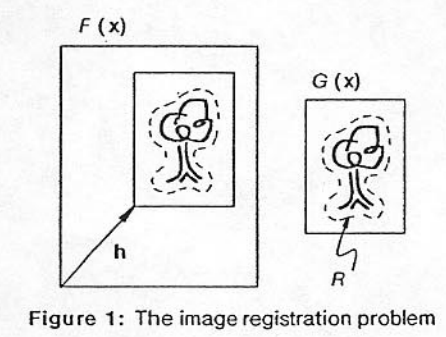F(x+h)与G(x)差异的典型度量方式有：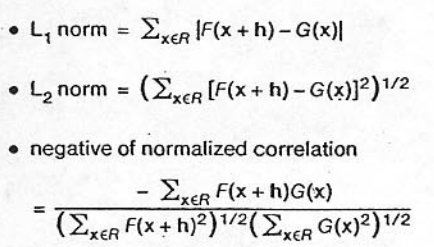首先，我们来看看一维情况下的图像配准问题，如下图，我们希望找到F(x)和G(x)=F(x+h)的水平视差h：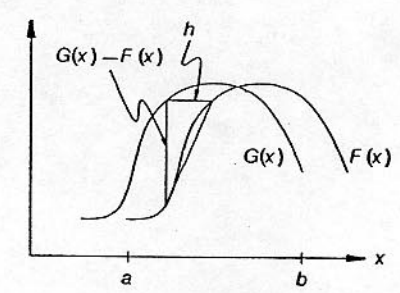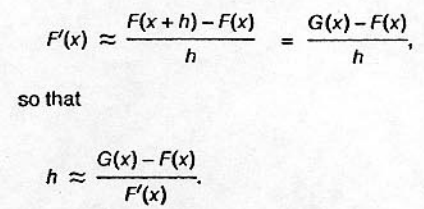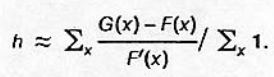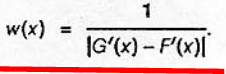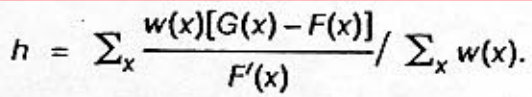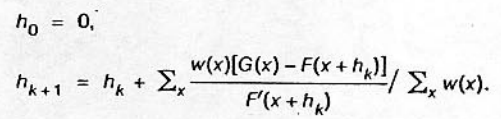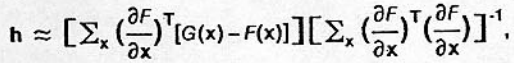Jianbo Shi和Carlo Tomasi两人在1994的CVPR上发表了篇论文：Good Features To Track中该该算子进行了扩展，通过一个仿射运动域来表示前面提到的h，则J(x)和I(x)表示为：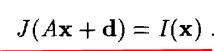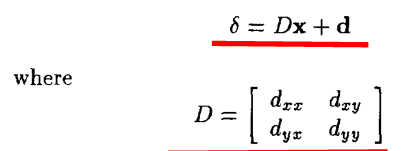由于上式J(Ax+d)=I(x)不能很好的满足，因此求解A和d一般是通过对下式进行最小化求解：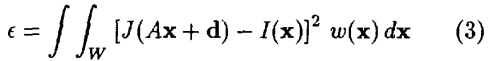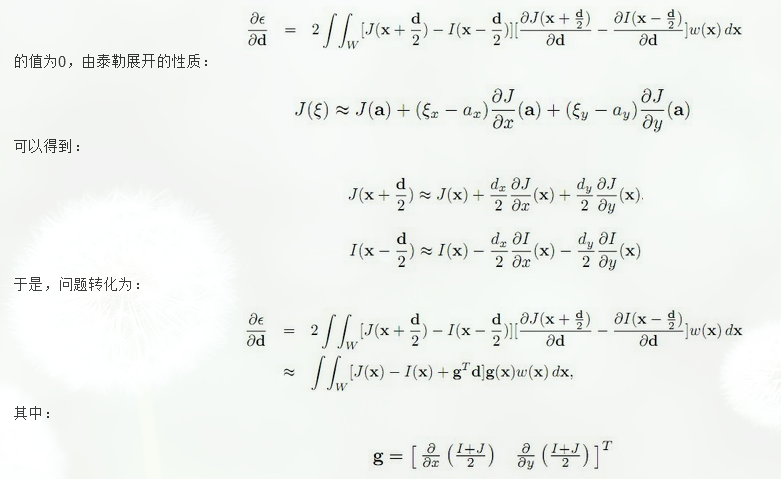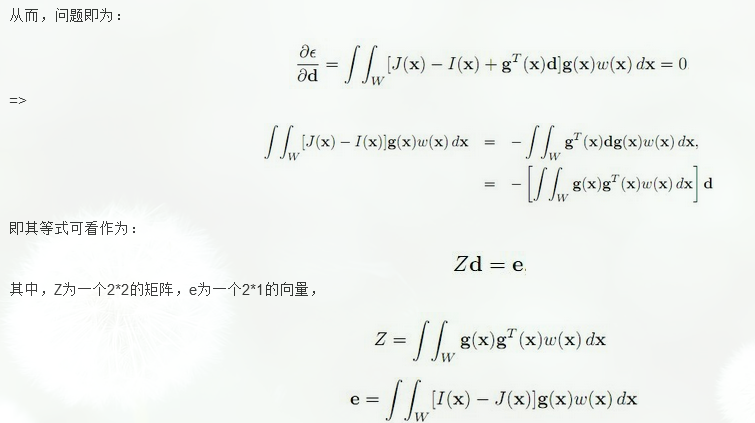KLT是一种优秀的算子，在目标跟踪系统中得到了广泛的应用。并且针对其速度的缺陷，现在也有基于GPU版本的加速KLT算法，具体的可以参考后面给出的参考资料。

1、KLT: An Implementation of the Kanade-Lucas-Tomasi Feature Tracker：http://www.ces.clemson.edu/~stb/klt/

#### 给我老师的人工智能教程打call！http://blog.csdn.net/jiangjunshow2018-09-27 09:12:36 peach_orange 阅读数 872
• ###### MATLAB图像处理

全面系统的学习MATLAB在图像处理中的应用

19898 人正在学习 去看看 魏伟

# 图像处理之特征提取：HOG特征简单梳理

## 转载自：https://blog.csdn.net/coming_is_winter/article/details/72850511

HOG 方向梯度直方图，这里分解为方向梯度与直方图。

## 一、方向梯度

在单变量的实值函数的情况，梯度只是导数，或者，对于一个线性函数，也就是线的斜率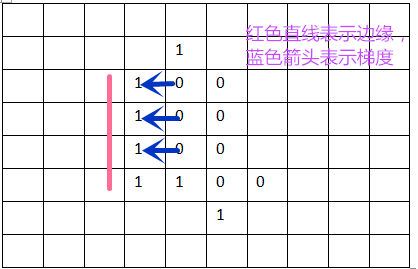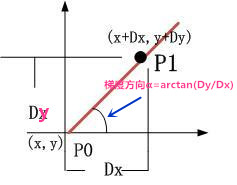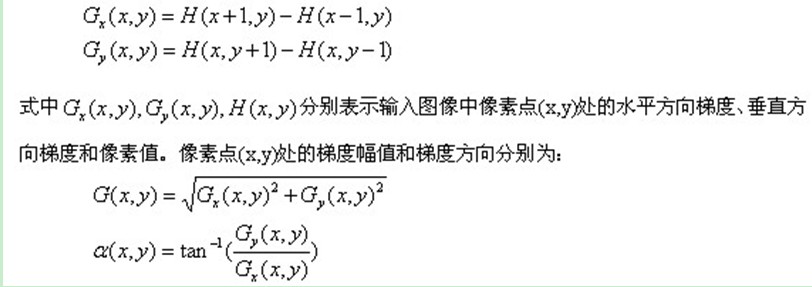## 二、直方图

直方图是一个图像处理中用的比较多的概念，想深入了解，可以度娘一下。

## 三、方向梯度直方图HOG的提取

方向梯度直方图为图像局部区域的梯度特征量统计，我们为什么要提取这个东东呢？

HOG主要应用于行人检测方面，以行人照片为例。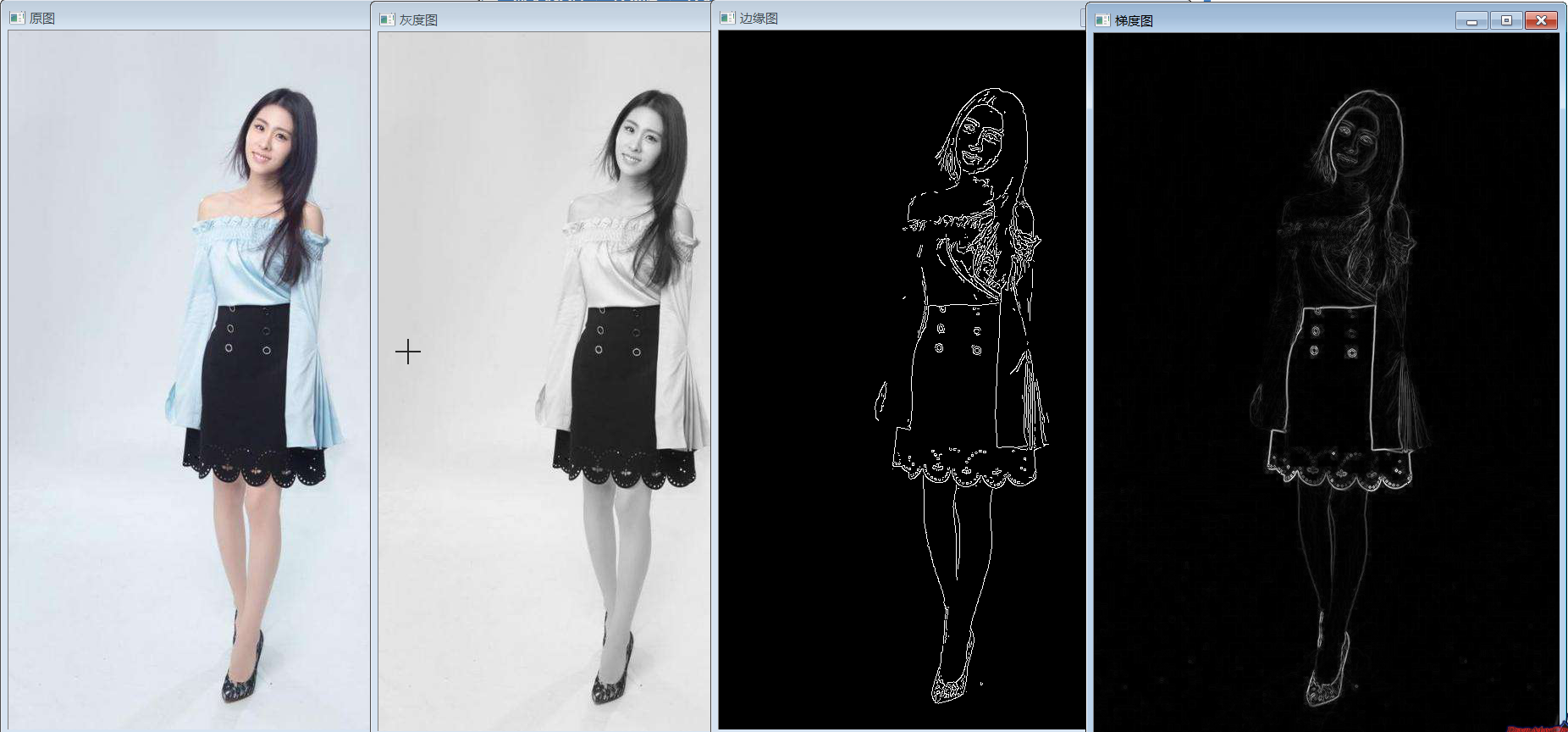上图是一张行人图的四种表示方式，原三色图，灰度图，边缘图，梯度图，人脑根据前期学习与先验知识很容易理解到图像中包含着一个行人，并可以根据一定情况将其从图像中抠选出来，但计算机是怎么思考的呢？怎样让计算机理解以上图像中包含的是一个行人呢？前三个图像现在情况不适用，所以选取梯度图，现在的梯度图同样也是人脑处理理解的平面结果，计算机是办不到的，需要将直观地的梯度图像转换成一种计算机容易理解的数据特征语言。

对于64*128的图像而言，每8*8的像素组成一个cell，每2*2个cell组成一个块，以8个像素为步长，那么，水平方向将有7个扫描窗口，垂直方向将有15个扫描窗口。也就是说，64*128的图片，总共有36*7*15=3780个特征。这里截取梯度图的一部分画图进行理解，尺寸与比例并不精确。单独将其中一个8*8的小格拿出来，方向梯度中指的方向范围为2π，360°，为了画直方图我们还需要选取合适的组距也就是bin，这里组距选取2π/9，也就是最后的直方图组数为9。下图为8*8像素的cell对应的方向梯度（未全部画出，共有8*8=64个）。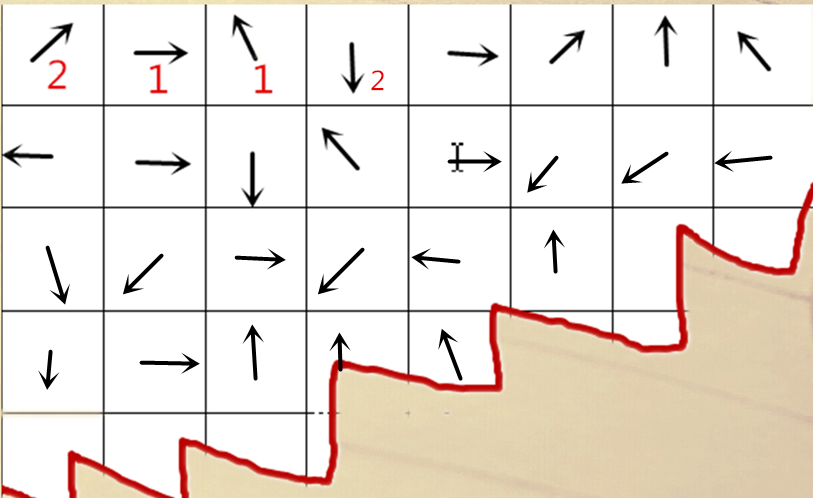将上面的64个方向梯度，按着直方图的参数设置进行画图，其中梯度的大小在统计数量中呈线性关系，比如梯度大小为2，则直方图对应增加2个单位，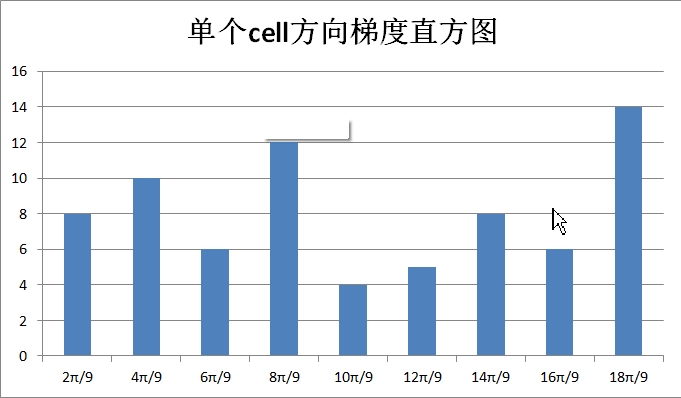把上图中单个cell对应的方向直方图转换为单维向量，也就是按规定组距对对应方向梯度个数进行编码，（8,10,6,12,4,5,8,6,14），得到单个cell的9个特征，每个block（扫描窗口）包含2*2个cell也就是2*2*9=36个特征，一个64*128大小的图像最后得到的特征数为36*7*15=3780个。这样将一幅直观的梯度图通过分解提取变为计算机容易理解的特征向量。

以上工作为HOG提取的主要内容，最后得到对应的行人的由方向梯度直方图HOG提取到的特征向量，但是计算机还是不知道这个数据数组代表了什么意思，什么时候这组向量代表行人，什么时候代表其他东西，怎样train，最后通过不断地学习，而后在检测积累的基础上对对未知图像检测识别有没有行人呢？那就是后一步SVM要做的事了。

zouxy09   http://blog.csdn.net/zouxy09/article/details/7929348/    目标检测的图像特征提取之（一）HOG特征

--------------------- 本文来自 Coming_is_winter 的CSDN 博客 ，全文地址请点击：https://blog.csdn.net/coming_is_winter/article/details/72850511?utm_source=copy

2018-06-28 17:12:28 qq_28193895 阅读数 11125
• ###### MATLAB图像处理

全面系统的学习MATLAB在图像处理中的应用

19898 人正在学习 去看看 魏伟

## Susan

SUSA（Smallest Univalue Segment Assimilating Nucleus）算子是一种高效的边缘和角点检测算子，并且具有结构保留的降噪功能

### 原理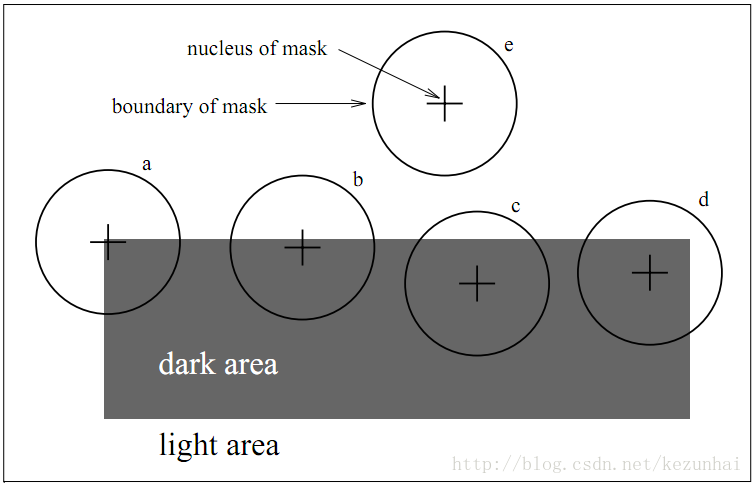## 实现步骤

1. 利用圆形模板遍历图像，计算每点处的USAN值
2. 设置一阈值g，一般取值为1/2(Max(n)）， 也即取值为USAN最大值的一半，进行阈值化，得到角点响应
3. 使用非极大值抑制来寻找角点

## 优缺点

• 完全不涉及梯度的运算，因此其抗噪声能力很强，运算量也比较小
• SUSAN算子还是一个各向同性的算子
• 图像的对比度较大，则可选取较大的t值，而图像的对比度较小，则可选取较小的t值
• 不仅具有很好的边缘检测性能；而且对角点检测也具有很好的效果。

## FAST

FAST( Features from Accelerated Segment Test)由Rosten等人在SUSAN角点特征检测方法的基础上利用机器学习方法提出.

### 原理

#### 分割测试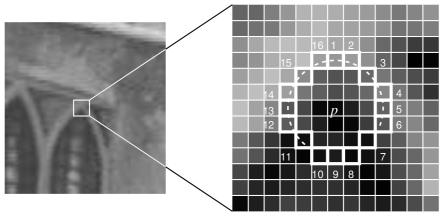t是一个阈值（默认取值为10，不同场景取值有差异），${I}_{p}$$I_p$表示的是中心像素的像素值，${I}_{p\to x}$$I_{p{\rightarrow}x}$表示的是圆形模板中的像素值。统计圆形区域中d或b的个数，只要d或b出现的次数大于n（（当是12点分割测试角点检测时，n=12；当是9点时，则n=9），那么该点就被认为是候选角点。

${S}_{p\to x}=\left\{\begin{array}{ll}d,{I}_{p\to x}<{I}_{p}-t,& \text{dark}\\ s,{I}_{p}-t<{I}_{p\to x}<{I}_{p}+t,& \text{similiar}\\ b,{I}_{p\to x}>{I}_{p}+t,& \text{brighter}\end{array}$

#### ID3训练

$H\left(P\right)=\left(c+{c}^{-}\right){\mathrm{log}}_{2}\left(c+{c}^{-}\right)-c{\mathrm{log}}_{2}c-{c}^{-}{\mathrm{log}}_{2}{c}^{-}$

$H\left(P\right)-H\left({P}_{d}\right)-H\left({P}_{s}\right)-H\left({P}_{b}\right)$

#### 非极大值抑制

$V=max\left(\sum _{xϵ{S}_{bright}}|{I}_{p\to x}-{I}_{p\to }|-t,\sum _{xϵ{S}_{dark}}|{I}_{p\to x}-{I}_{p\to }|-t\right)$

### 实现步骤

1. 对固定半径圆上的像素进行分割测试，通过逻辑测试可以去处大量的非特征候选点；
2. 基于分类的角点特征检测，利用ID3 分类器根据16个特征判决候选点是否为角点特征，每个特征的状态为一1，0，1。
3. 利用非极大值抑制进行角点特征的验证。

### 优缺点

• 计算速度快，可以应用于实时场景中
• 容易受到噪声影响，阈值t的影响也较大

## BRIEF

Brief为特征描述子，对已检测到的特征点进行描述，是一种二进制编码描述子

### 实现步骤

1. 为减少噪声干扰，先对图像进行高斯滤波（方差2，窗口9x9）
2. 以特征点为中心，取SxS的邻域大窗口。在大窗口中随机选取(有5种经典方法)一对（两个）5x5的子窗口，比较子窗口内的像素和（可用积分图像完成），进行二进制赋值。（一般S=31），其中$p\left(x\right)$$p(x)$,$p\left(y\right)$$p(y)$分别为所在5x5子窗口的像素和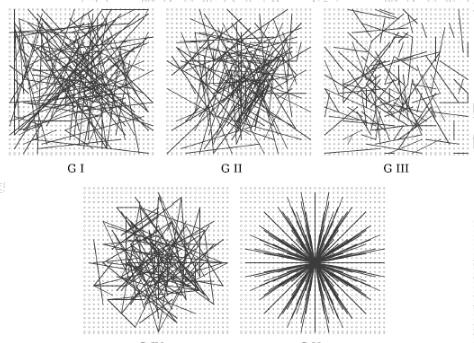1. 在大窗口中随机选取N对子窗口，重复步骤2的二进制赋值，形成一个二进制编码，这个编码就是对特征点的描述，即特征描述子。（一般N=256）构造一个512个bit的BRIEF，就需要512对[x,y]，且需要注意，它们是有序的，每次计算位置都相同，否则影响最终结果。也就说说，一旦选定了512对[x,y]，那么，无论是提取特征，还是匹配特征，都要按照这512对进行计算。
2. 汉明距离进行配对。两个特征编码对应bit位上相同元素的个数小于128的，一定不是配对的。一幅图上特征点与另一幅图上特征编码对应bit位上相同元素的个数最多的特征点配成一对。

### 优缺点

• 抛弃了传统的用梯度直方图描述区域的方法，改用检测随机响应，大大加快了描述子建立速度
• 生成的二进制描述子便于高速匹配，计算Hamming距离只需通过异或操作加上统计二进制编码中“1”的个数的操作，这些通过底层的运算即可实现
• 缺点很明显就是旋转不变性较差

## DoG

DoG算子是由Lowe D.G.提出的，对噪声、尺度、仿射变化和旋转等具有很强的鲁棒性，能够提供更丰富的局部特征信息

### 原理

#### 图像金字塔多分辨率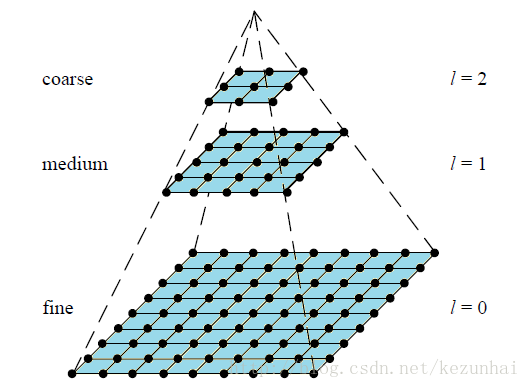#### 高斯平滑滤波

$G\left({x}_{i},{y}_{i},\sigma \right)=\frac{1}{2\pi {\sigma }^{2}}exp\left(-\frac{\left(x-{x}_{i}{\right)}^{2}+\left(y-{y}_{i}{\right)}^{2}}{2{\sigma }^{2}}\right)$

$L\left(x,y,\sigma \right)=G\left(x,y,\sigma \right)\ast I\left(x,y\right)$

#### 多尺度与多分辨率

1. 尺度空间表达是由不同高斯核平滑卷积得到，在所有尺度上有相同的分辨率
2. 金字塔多分辨率表达每层分辨率减少固定比率

#### DoG金字塔

DoG（Difference of Gaussian）其实是对高斯拉普拉斯(LoG)的近似，在某一尺度上的特征检测可以通过对两个相邻高斯尺度空间的图像相减，得到DoG的响应值图像$D\left(x,y,\sigma \right)$$D(x,y,\sigma)$，这比直接计算LoG效率更高。

• 对原图进行相邻尺度的高斯滤波
${g}_{1}={G}_{{\sigma }_{1}}\left(x,y\right)\ast f\left(x,y\right)$

${g}_{2}={G}_{{\sigma }_{2}}\left(x,y\right)\ast f\left(x,y\right)$
• 将上面的滤波得到的两幅图像相减：
${g}_{1}\left(x,y\right)-{g}_{2}\left(x,y\right)={G}_{{\sigma }_{1}}\ast f\left(x,y\right)-{G}_{{\sigma }_{2}}\ast f\left(x,y\right)=\left({G}_{{\sigma }_{1}}-{G}_{{\sigma }_{2}}\right)\ast f\left(x,y\right)=DoG\ast f\left(x,y\right)$
• 将公式简化写法：

$D\left(x,y,\sigma \right)=\left(G\left(x,y,k\sigma \right)-G\left(x,y,\sigma \right)\right)⨂I\left(x,y\right)$
$=L\left(x,y,k\sigma \right)-L\left(x,y,\sigma \right)$

• 构造高斯金字塔
为了得到DoG图像，首先要构造高斯金字塔，高斯金字塔在多分辨率金字塔的基础上加入了高斯滤波，也就是对金字塔每层图像采用不同的参数sigma进行了高斯卷积，使得金字塔的每层有多张图片组成为一个Octave，每组有多张（也叫层interval）图像。每个Octave是由同一大小的图像，经过不同sigma高斯滤波得到的，而Interval则表示的是同一个sigma高斯滤波的图像。另外，降采样时，金字塔上边一组图像的第一张图像（最底层的一张）是由前一组（金字塔下面一组）图像的倒数第三张隔点采样得到，图像表示如下：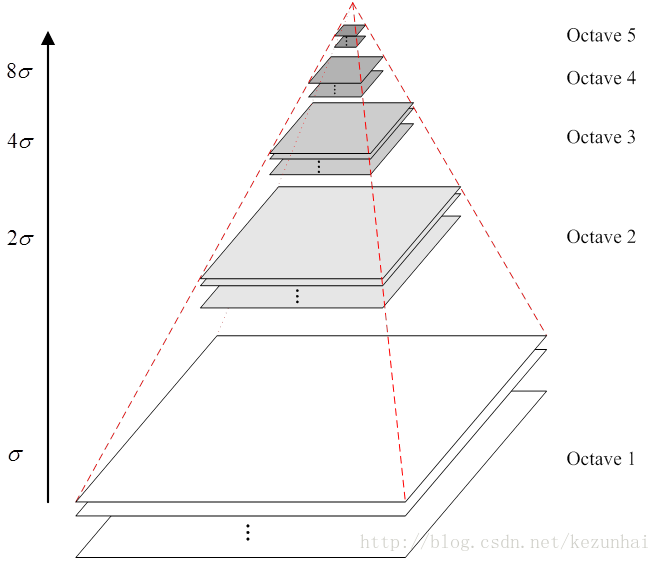• 求DoG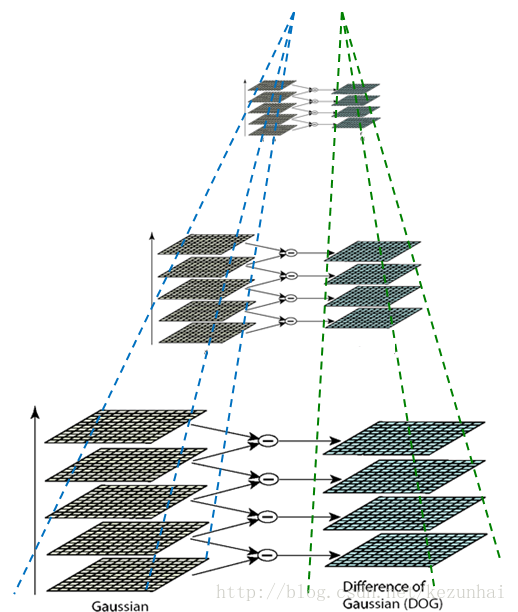#### 求角点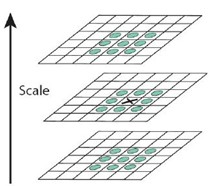X标记当前像素点，绿圈标记邻接像素点，用这个方式，最多检测相邻尺度的26个像素点。如果它是所有邻接像素点的最大值或最小值点，则X被标记为特征点，如此依次进行，则可以完成图像的特征点提取。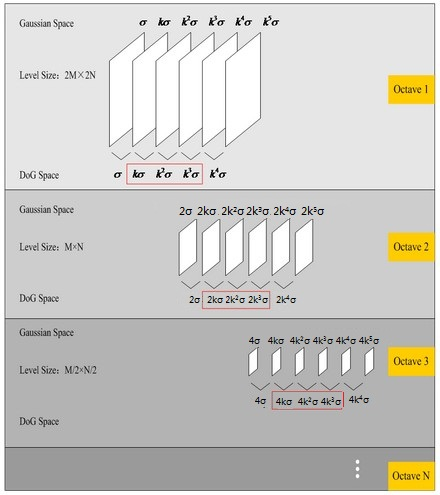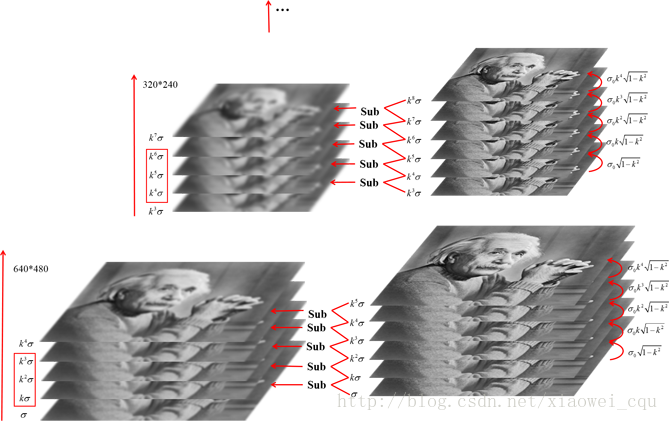• 具有尺度不变特性，抗旋转
• 计算量大

## RANSC特征点筛选

RANSAC算法的基本假设是样本中包含正确数据(inliers，可以被模型描述的数据)，也包含异常数据(outliers，偏离正常范围很远、无法适应数学模型的数据)，即数据集中含有噪声。这些异常数据可能是由于错误的测量、错误的假设、错误的计算等产生的。同时RANSAC也假设，给定一组正确的数据，存在可以计算出符合这些数据的模型参数的方法。

### 实现步骤

• 假定模型（如直线方程），并随机抽取Nums个（以2个为例）样本点，对模型进行拟合
• 由于不是严格线性，数据点都有一定波动，假设容差范围为：sigma，找出距离拟合曲线容差范围内的点，并统计点的个数
• 重新随机选取Nums个点，重复第一步~第二步的操作，直到结束迭代
• 每一次拟合后，容差范围内都有对应的数据点数，找出数据点个数最多的情况，就是最终的拟合结果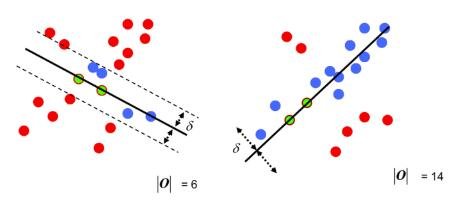### 优缺点

• 最小二乘法适用于有效数据占大多数，无效数据少的情况，是从一个整体误差最小的角度去考虑。RANSC基于假设寻找最优。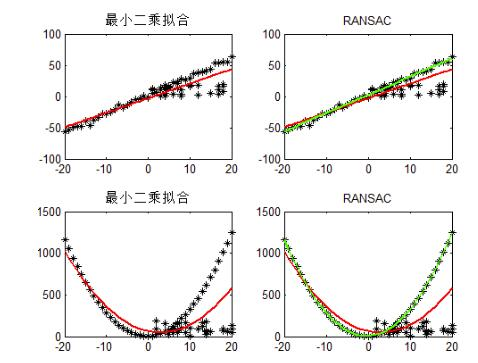## Harris角点检测

### 原理

• 数学刻画

$E\left(U,V\right)=\sum _{x,y}w\left(x,y\right)\left[I\left(x+u,y+v\right)-I\left(x,y\right){\right]}^{2}$

式中，$\left[u,v\right]$$[u,v]$是窗口的偏移量，$w\left(x,y\right)$$w(x,y)$是窗口的权重系数。一般可用高斯二维函数。

• 表达式演化-泰勒展开

$f\left(x+u,y+v\right)\approx f\left(x,y\right)+u{f}_{x}\left(x,y\right)+v{f}_{y}\left(x,y\right)$

$\begin{array}{}\text{(1)}& \sum \left[I\left(x+u,y+v\right)-I\left(x,y\right){\right]}^{2}& \approx \sum \left[I\left(x,y\right)+u{I}_{x}+v{I}_{y}-I\left(x,y\right){\right]}^{2}\text{(2)}& & =\sum {u}^{2}{{I}_{x}}^{2}+2uv{I}_{x}{I}_{y}+{v}^{2}{{I}_{y}}^{2}\text{(3)}& & =\sum \left[\begin{array}{cc}u& v\end{array}\right]\left[\begin{array}{cc}{{I}_{x}}^{2}& {I}_{x}{I}_{y}\\ {I}_{x}{I}_{y}& {{I}_{y}}^{2}\end{array}\right]\left[\begin{array}{c}u\\ v\end{array}\right]\text{(4)}& & =\left[\begin{array}{cc}u& v\end{array}\right]\left(\sum \left[\begin{array}{cc}{{I}_{x}}^{2}& {I}_{x}{I}_{y}\\ {I}_{x}{I}_{y}& {{I}_{y}}^{2}\end{array}\right]\right)\left[\begin{array}{c}u\\ v\end{array}\right]\end{array}$

• $E\left(u,v\right)可更新为$$E(u,v)可更新为$
$E\left(u,v\right)\approx \left[\begin{array}{cc}u& v\end{array}\right]M\left[\begin{array}{c}u\\ v\end{array}\right]$

$M=\sum _{x,y}w\left(x,y\right)\left[\begin{array}{cc}{{I}_{x}}^{2}& {I}_{x}{I}_{y}\\ {I}_{x}{I}_{y}& {{I}_{y}}^{2}\end{array}\right]$

### 实现步骤

1. 对每一个像素计算自相关矩阵M
2. 构造角点性映射图

$M=\left[\begin{array}{cc}A& C\\ C& B\end{array}\right]$

$C\left(x,y\right)=det\left(M\right)-k\left(trace\left(M\right){\right)}^{2}$

$det\left(M\right)={\lambda }_{1}{\lambda }_{2}=AB-{C}^{2}$

$trace\left(M\right)={\lambda }_{1}+{\lambda }_{2}=A+B$

3. 阈值化，对得到的$C\left(x,y\right)进行阈值分割$$C(x,y)进行阈值分割$

4. 非极大值抑制

### 优缺点

• 针对Moravec算子的不足进行了改进，提高了特征点的检测率
• Harris算子计算量大，对尺度很敏感，不具有尺度不变性
• Harris对特征点的定位也不是很精确
• Harris也是各向异性的，对噪声敏感。